Last updated: 2018-05-24

• R Markdown file: up-to-date

Great! Since the R Markdown file has been committed to the Git repository, you know the exact version of the code that produced these results.

• Environment: empty

Great job! The global environment was empty. Objects defined in the global environment can affect the analysis in your R Markdown file in unknown ways. For reproduciblity it’s best to always run the code in an empty environment.

• Seed: set.seed(1)

The command set.seed(1) was run prior to running the code in the R Markdown file. Setting a seed ensures that any results that rely on randomness, e.g. subsampling or permutations, are reproducible.

• Session information: recorded

Great job! Recording the operating system, R version, and package versions is critical for reproducibility.

• Repository version: 336a8b6

Great! You are using Git for version control. Tracking code development and connecting the code version to the results is critical for reproducibility. The version displayed above was the version of the Git repository at the time these results were generated.

Note that you need to be careful to ensure that all relevant files for the analysis have been committed to Git prior to generating the results (you can use wflow_publish or wflow_git_commit). workflowr only checks the R Markdown file, but you know if there are other scripts or data files that it depends on. Below is the status of the Git repository when the results were generated:

Ignored files:
Ignored:    .sos/
Ignored:    data/.sos/
Ignored:    gtex6_workflow_output/
Ignored:    workflows/.ipynb_checkpoints/
Ignored:    workflows/.sos/


Note that any generated files, e.g. HTML, png, CSS, etc., are not included in this status report because it is ok for generated content to have uncommitted changes.
Expand here to see past versions:
File Version Author Date Message
Rmd 336a8b6 Peter Carbonetto 2018-05-24 wflow_publish(“Fig.ExpressionAnalysis.Rmd”)
Rmd ae61358 Peter Carbonetto 2018-05-24 wflow_publish(“Fig.ExpressionAnalysis.Rmd”)
Rmd e8c6f6f Peter Carbonetto 2018-05-24 Working on revisions to Fig.ExpressionAnalysis.Rmd.
Rmd 5ed6715 Peter Carbonetto 2018-05-24 Moved AvgExpr..csv and working on changes to Fig.ExpressionAnalysis.Rmd analysis.
Rmd 80f285f Gao Wang 2017-09-20 Update figures
html 80f285f Gao Wang 2017-09-20 Update figures

In this analysis, we assess whether tissue-specific eQTLs we identified can be explained by tissue-specific expression. Specifically, we take genes with tissue-specific eQTLs, and examine the distribution of expression in the eQTL-affected tissue relative to expression in other tissues.

## Load data and MASH results

In the next code chunk, we load some GTEx summary statistics (average gene expression values and z-scores), as well as some of the results generated from the MASH analysis of the GTEx data.

Expression is here defined as median across individuals of the log Reads per Kilobase Mapped (RPKM).

data <- read.csv("../data/AvgExpr.csv.gz",header = TRUE)
maxz <- out$test.z qtl.names <- sapply(1:length(rownames(maxz)), function(x) unlist(strsplit(rownames(maxz)[x], "[_]"))[]) rownames(data) <- data[,1] expr.data <- data[,-1] out <- readRDS(paste("../output/MatrixEQTLSumStats.Portable.Z.coved.K3.P3", "lite.single.expanded.V1.posterior.rds",sep = ".")) pmash <- out$posterior.means
lfsr  <- out$lfsr colnames(lfsr) <- colnames(pmash) <- colnames(maxz) rownames(lfsr) <- rownames(pmash) <- rownames(maxz) expr.sort <- expr.data[rownames(expr.data)%in%qtl.names,] a <- match(qtl.names,rownames(expr.sort)) expr.sort <- expr.sort[a,] missing.tissues <- c(7,8,19,20,24,25,31,34,37) exp.sort <- expr.sort[,-missing.tissues] colnames(exp.sort) <- colnames(maxz) ## Define plotting functions Here we define a couple functions used to compare the densities and CDFs of gene expression levels. plot_tissuespecifictwo = function(tissuename,lfsr,ymax,curvedata,title, thresh=0.05,subset=1:44,exclude=0.01) { index_tissue=which(colnames(lfsr) %in% tissuename); ybar=title # Create a matrix showing whether or not lfsr satisfies threshold. sigmat = lfsr <= thresh; sigs=which(rowSums(sigmat[,index_tissue,drop=FALSE])==length(tissuename) & rowSums(sigmat[,-index_tissue,drop=FALSE])==0) norm.spec=density(curvedata[sigs,index_tissue]) norm.other=density(curvedata[-sigs,index_tissue]) xmin=min(norm.spec$x,norm.other$x)-1 ymin=min(norm.spec$y,norm.other$y) xmax=max(norm.spec$x,norm.other\$x)+1

plot(norm.other,xlim = c(xmin,xmax),ylim=c(0,ymax),
col="black",main=tissuename,xlab="log(RPKM)")

lines(norm.spec,col="red")
legend("right",legend = c("All Genes","Tissue Specific"),
col=c("black","red"),pch=c(1,1))
}

plot_tissuespecificthree = function(tissuename,lfsr,ymax,curvedata,title,
thresh=0.05,subset=1:44,exclude=0.01) {
index_tissue=which(colnames(lfsr) %in% tissuename);
ybar=title

# Create a matrix showing whether or not lfsr satisfies threshold.
sigmat = lfsr <= thresh
sigs=which(rowSums(sigmat[,index_tissue,drop=FALSE])==length(tissuename) &
rowSums(sigmat[,-index_tissue,drop=FALSE])==0)
norm.spec=ecdf(curvedata[sigs,index_tissue])
norm.other=ecdf(curvedata[-sigs,index_tissue])
plot(norm.other,ylim=c(0,ymax),
col="black",main=tissuename,xlab="log(RPKM)")

lines(norm.spec,col="red",cex=0.1)
legend("right",legend = c("All Genes","Tissue Specific"),
col=c("black","red"),pch=c(1,1))
} 

## Distribution of expression levels in testis

The two plots below compare the densities and cumulative distribution functions of the expression levels for all genes (black), and for genes identified as having a “tissue-specific” eQTL (red) in testis.

plot_tissuespecifictwo(tissuename = "Testis",lfsr = lfsr,
curvedata = log(exp.sort),title = "Test",
thresh = 0.05 ,ymax=0.5)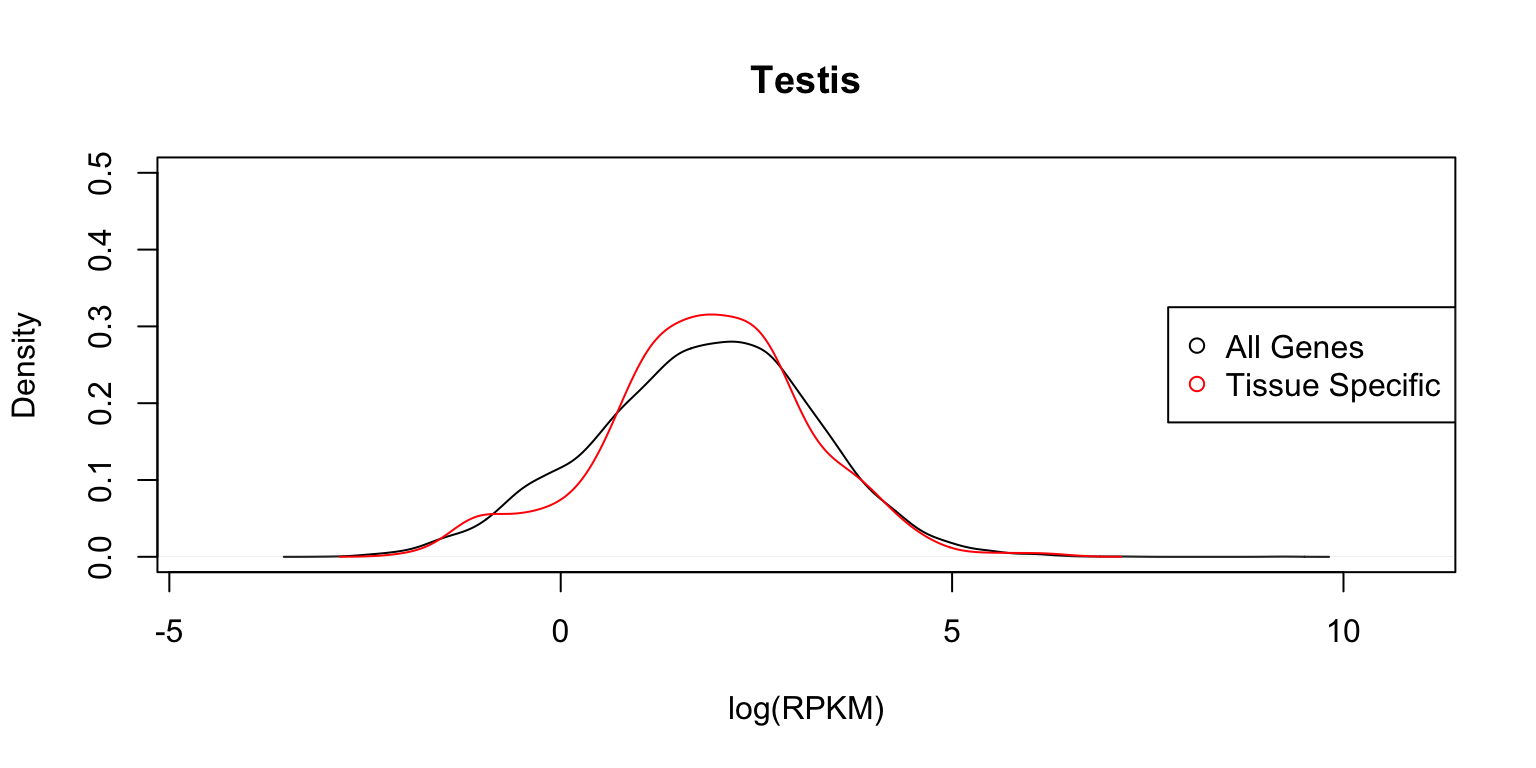plot_tissuespecificthree(tissuename = "Testis",lfsr = lfsr,
curvedata = log(exp.sort),title = "Test",
thresh = 0.05 ,ymax=1)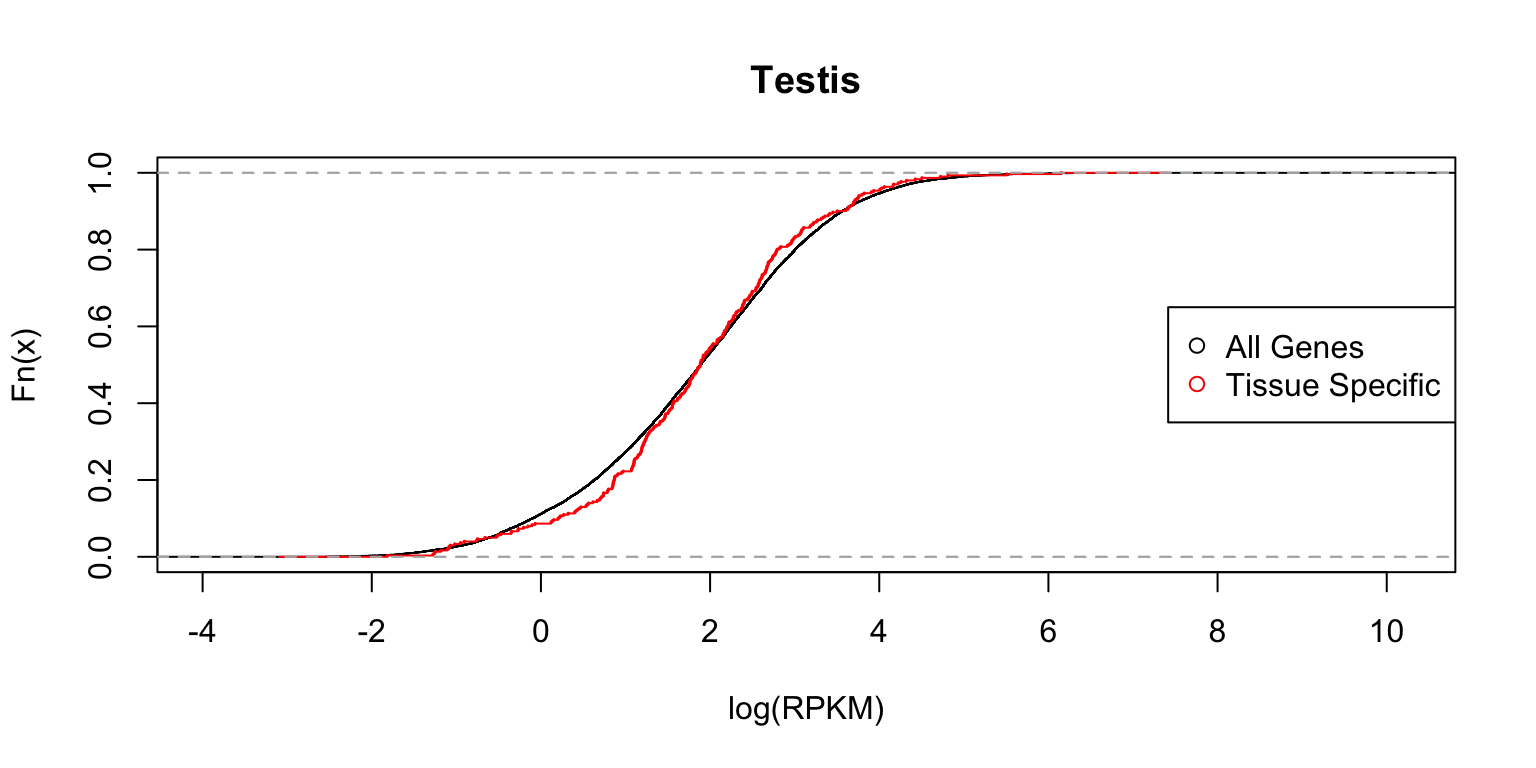The distribution functions are reasonably similar.

## Distribution of expression levels in thyroid

Next we show the same two plots for thyroid.

plot_tissuespecifictwo(tissuename = "Thyroid",lfsr = lfsr,
curvedata = log(exp.sort),title = "Test",
thresh = 0.05,ymax = 0.5)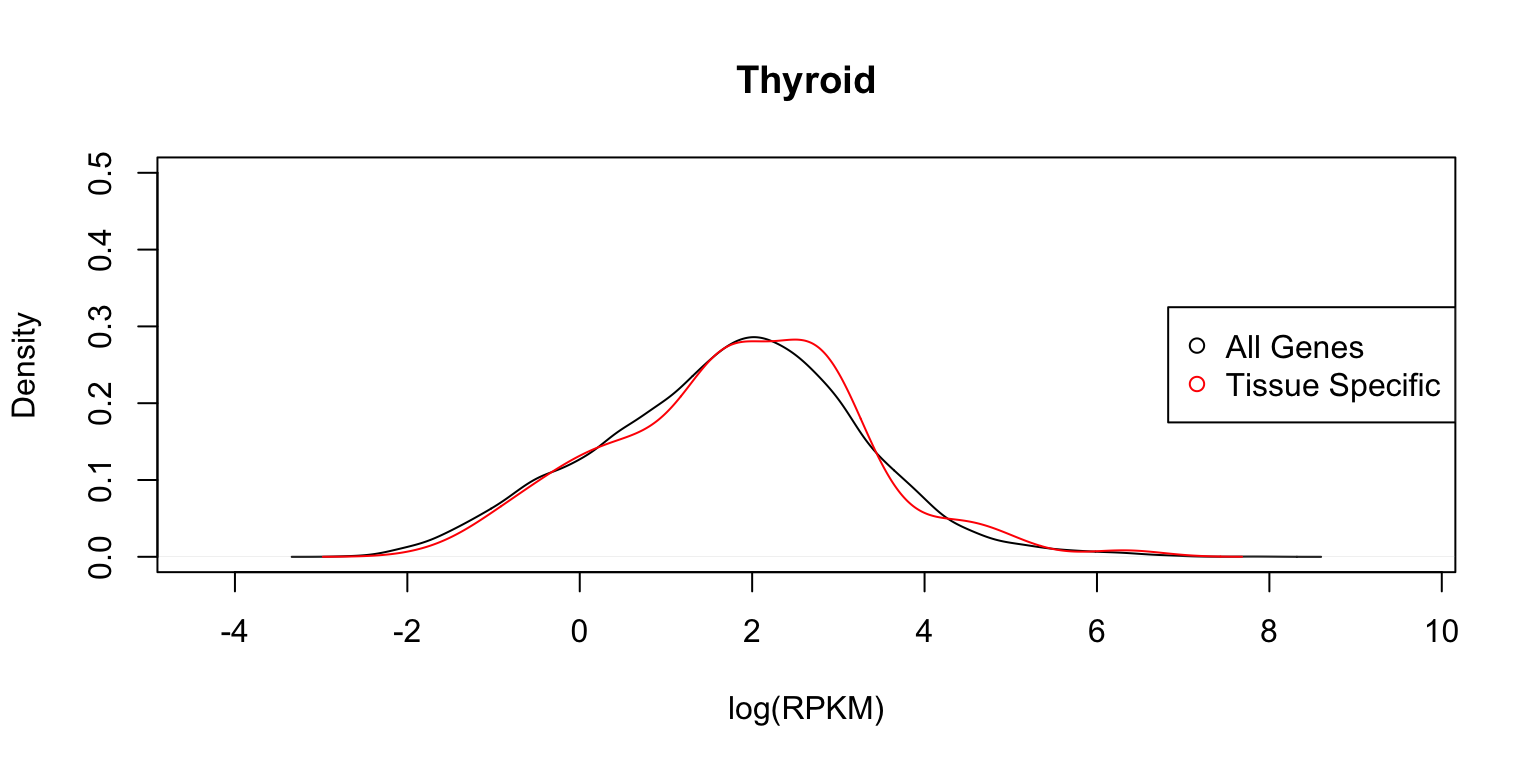plot_tissuespecificthree(tissuename = "Thyroid",lfsr = lfsr,
curvedata = log(exp.sort),title = "Test",
thresh = 0.05,ymax = 1)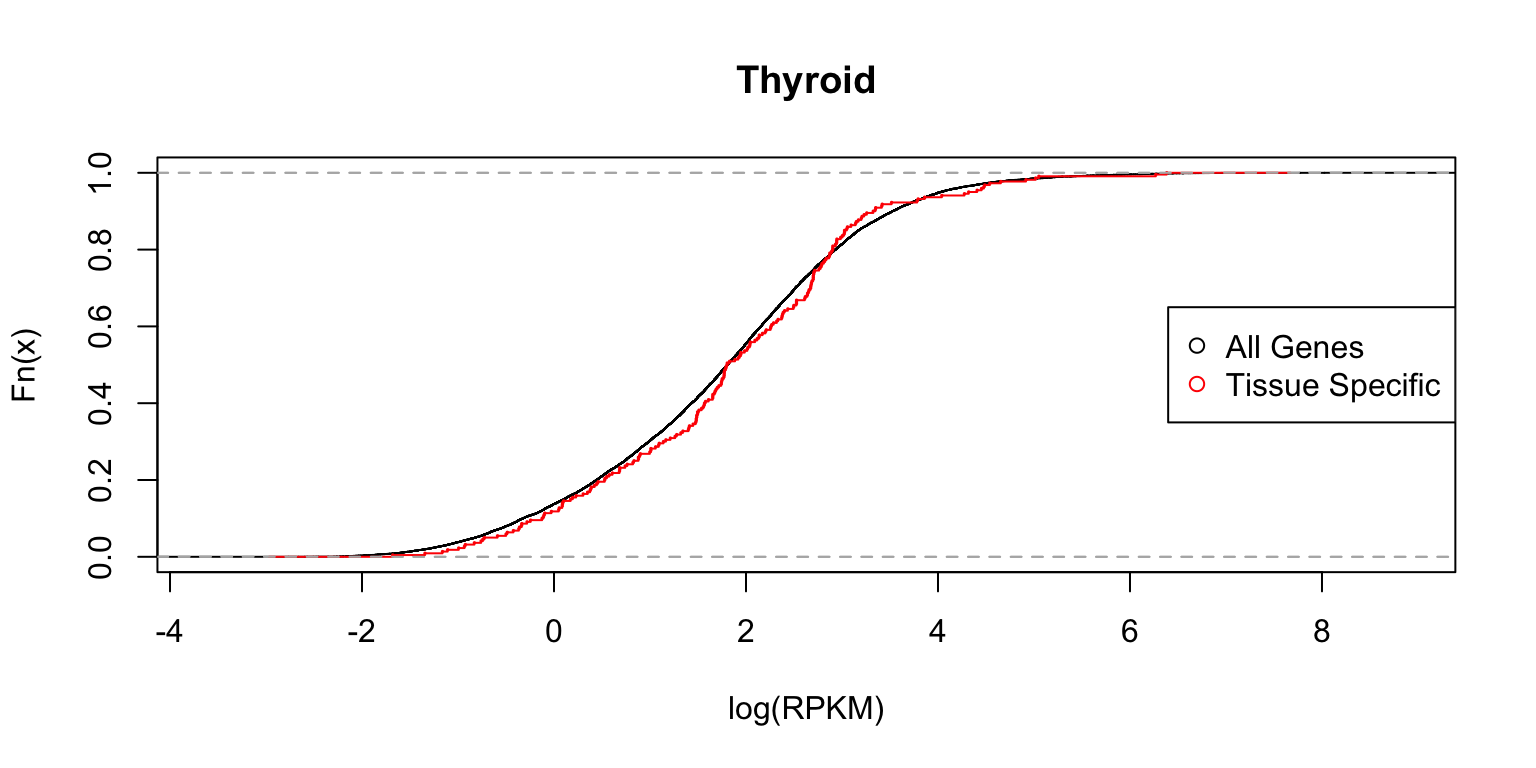## Distribution of expression levels in whole blood

Next, we look at the distributions in whole blood cells.

plot_tissuespecifictwo(tissuename = "Whole_Blood",lfsr = lfsr,
curvedata = log(exp.sort),title = "Test",
thresh = 0.05,ymax=0.5)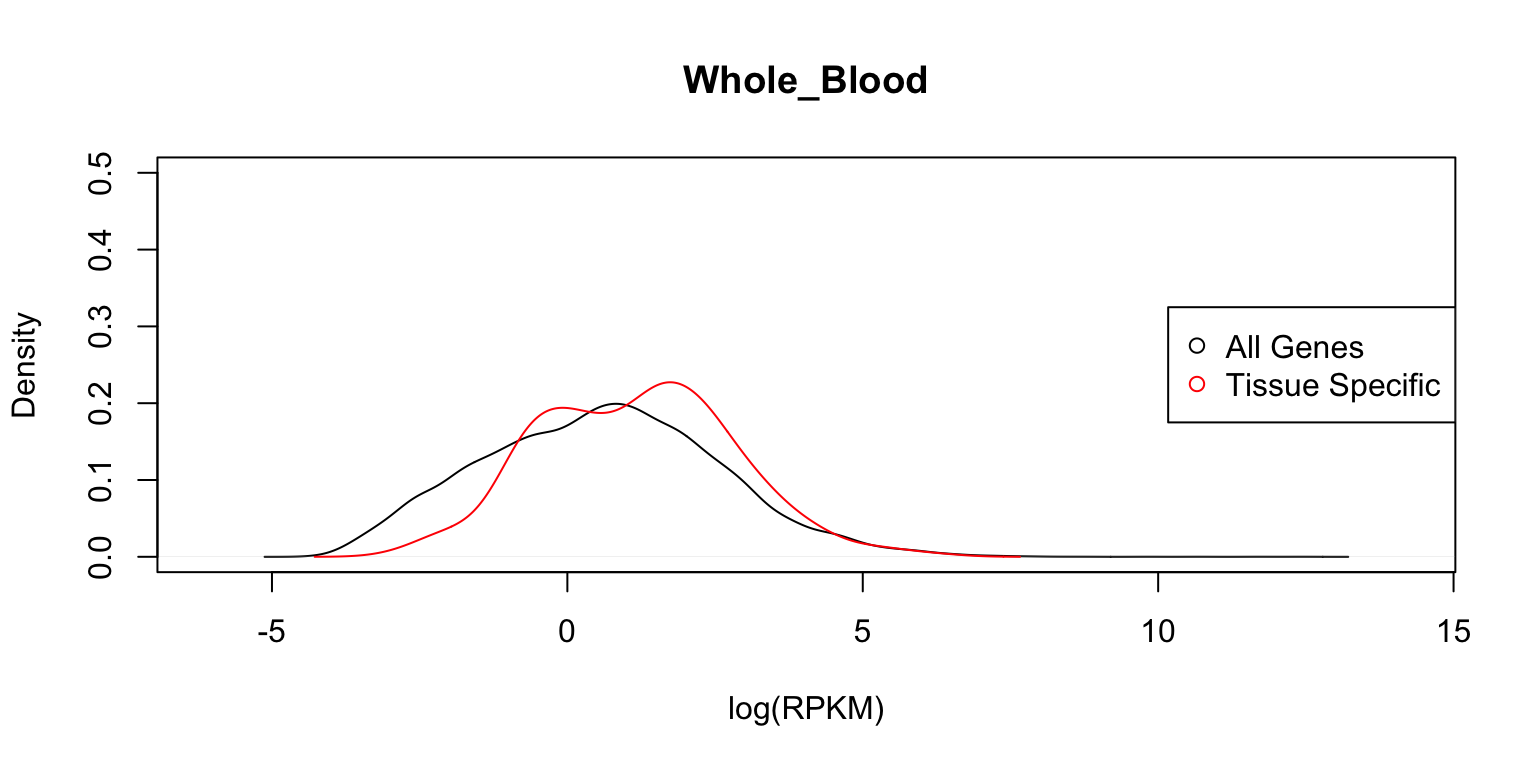plot_tissuespecificthree(tissuename = "Whole_Blood",lfsr = lfsr,
curvedata = log(exp.sort),title = "Test",
thresh = 0.05 ,ymax=1)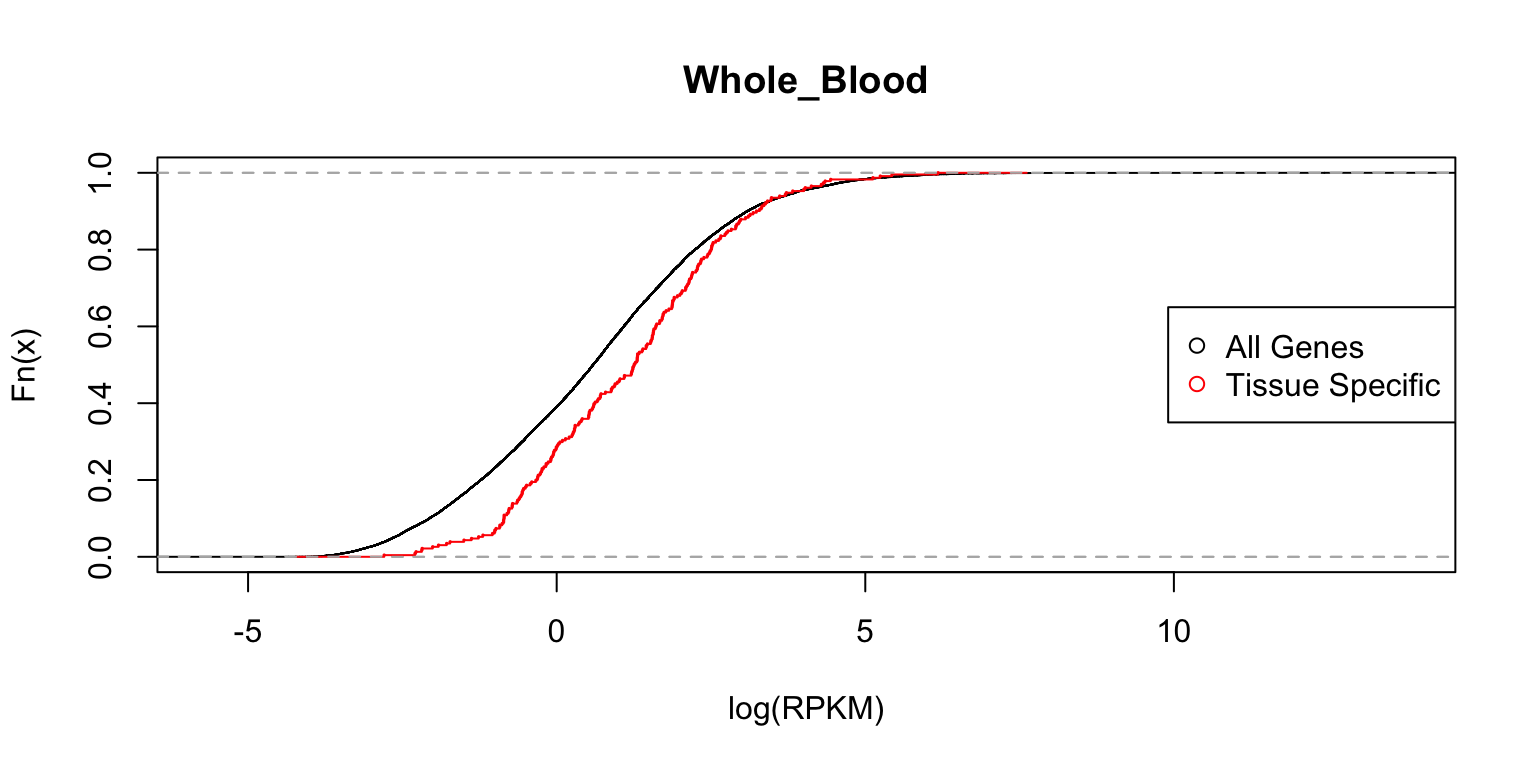## Distribution of expression levels in fibroblasts

Finally, we examine the gene expression distributions in fibroblasts.

plot_tissuespecifictwo(tissuename = "Cells_Transformed_fibroblasts",
lfsr = lfsr,curvedata = log(exp.sort),title = "Test",
thresh = 0.05,ymax=0.5)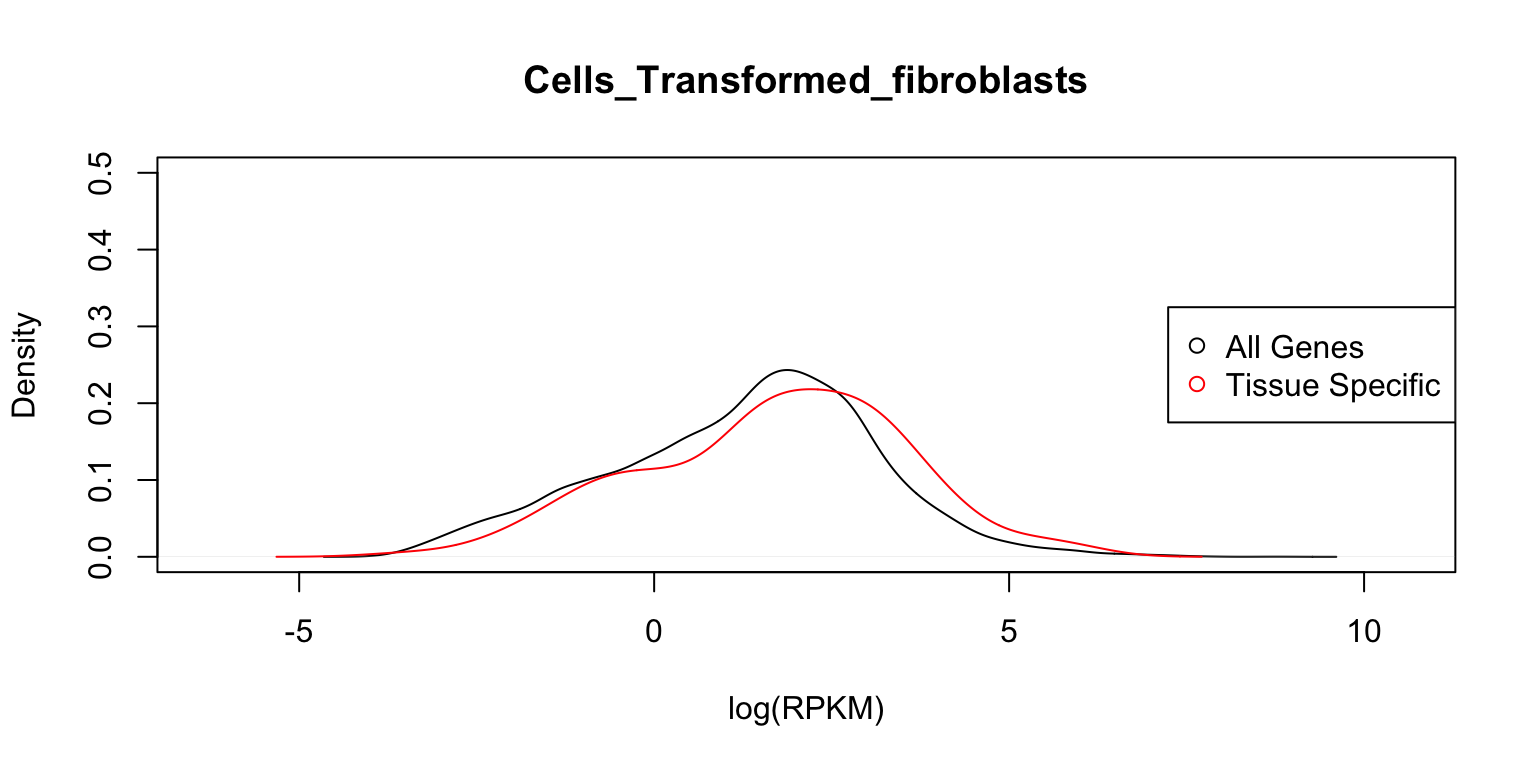plot_tissuespecificthree(tissuename = "Cells_Transformed_fibroblasts",
lfsr = lfsr,curvedata = log(exp.sort),title = "Test",
thresh = 0.05 ,ymax=1)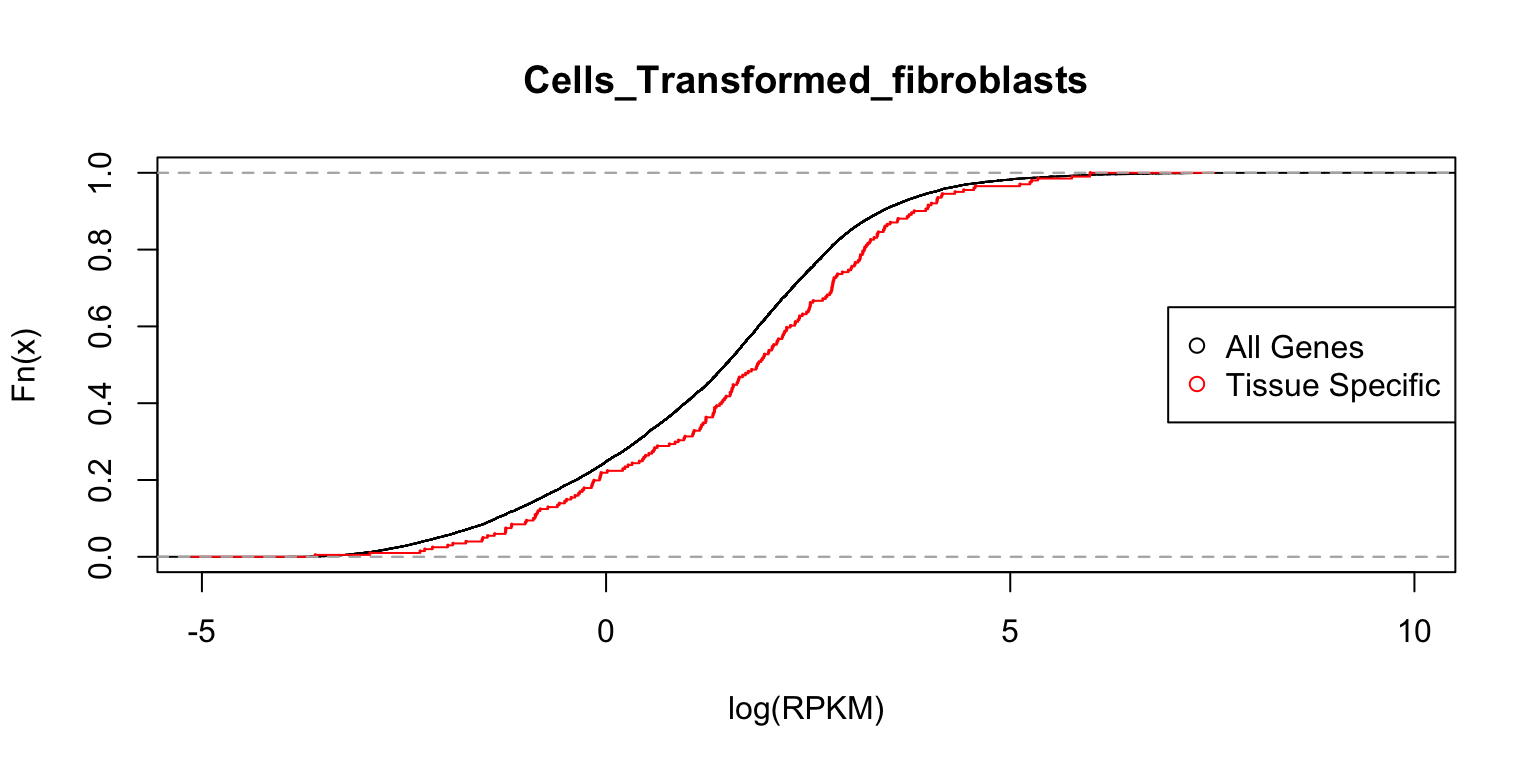In each case, the distribution functions are similar. This tells us that tissue-specific eQTLs are not simply reflecting tissue-specific expression.

## Session information

sessionInfo()
R version 3.4.3 (2017-11-30)
Platform: x86_64-apple-darwin15.6.0 (64-bit)
Running under: macOS High Sierra 10.13.4

Matrix products: default
BLAS: /Library/Frameworks/R.framework/Versions/3.4/Resources/lib/libRblas.0.dylib
LAPACK: /Library/Frameworks/R.framework/Versions/3.4/Resources/lib/libRlapack.dylib

locale:
 en_US.UTF-8/en_US.UTF-8/en_US.UTF-8/C/en_US.UTF-8/en_US.UTF-8

attached base packages:
 stats     graphics  grDevices utils     datasets  methods   base

loaded via a namespace (and not attached):
 workflowr_1.0.1.9000 Rcpp_0.12.16         digest_0.6.15
 rprojroot_1.3-2      R.methodsS3_1.7.1    backports_1.1.2
 git2r_0.21.0         magrittr_1.5         evaluate_0.10.1
 stringi_1.1.7        whisker_0.3-2        R.oo_1.21.0
 R.utils_2.6.0        rmarkdown_1.9        tools_3.4.3
 stringr_1.3.0        yaml_2.1.18          compiler_3.4.3
 htmltools_0.3.6      knitr_1.20          

This reproducible R Markdown analysis was created with workflowr 1.0.1.9000